• 要说清这个问题，先抛出另外一个问题：vector和普通的数组有什么区别？ 你可能会说：大家都知道，vector是动态数组~~ 没错！ 但是怎么个动态法？怎么实现的？ 好，先说一下普通数组，比如：int arr或者int *...
要说清这个问题，先抛出另外一个问题：vector和普通的数组有什么区别？
你可能会说：大家都知道，vector是动态数组~~
没错！  但是怎么个动态法？怎么实现的？
好，先说一下普通数组，比如：int arr或者int *arr = new int; 其实是你到内存里占了10个int大小的地方（空间）；
当然，你可以这样操作：int a = arr;   arr = 10;  这样编译器并不会报错，最多会警告你----你有越界行为。
也就是说，你一开始说好了，你只占10个大小的地方，后来你出尔反尔，又去访问或者操作第100个地方（为了说明问题，事实上是第101个），那么内存的“管理员”他没有不让你操作的权利，只是给你出示了“黄牌警告”，告诉你：你去操作吧，出了问题概不负责！！！        这就是数组越界问题，越界会造成未知的问题（程序崩溃等等），即你写的程序不受你控制了，或者不是你想要的结果（比如：你越界访问的地方是别人的地方，如果别人也没做“防护”，那么你修改了那个地方的值，就会让别人的会出现未知错误）。为了避免这个问题，那就老老实实的在那个10个大小的地方活动。
但是，在实际应用中，程序员的确有这样的需求：我开始是分配了10个大小的地方，但是我想给它后面添加一个或者给中间某个位置插入一个，变成连续11个数值。
我再描述一下这个需求：在一个连续的数组添加元素，还能保证新的数组仍然在内存连续，也就是说用下标可以访问（arr访问第11个元素）。
vector就实现了这样的功能，当然vector功能不止这一个，还有很多操作（push_back,insert等）。
那么，vector是怎么实现这样的功能的？可以参考《STL源码剖析》
我们以push_back()为例，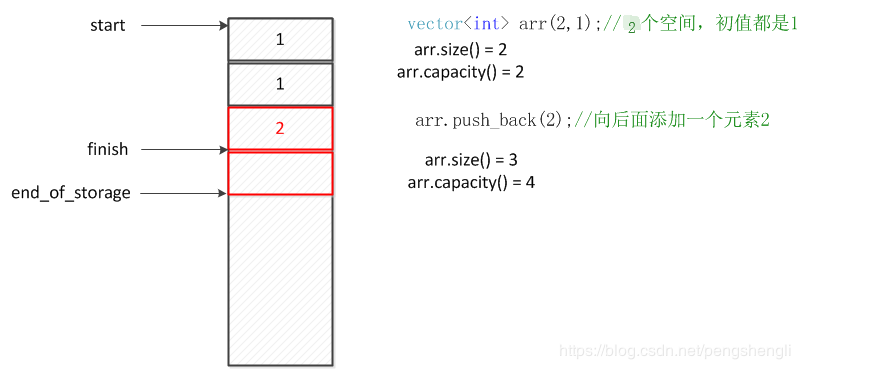其中size就是实际存入数据空间的大小，capacity是容量，即能容纳的数据个数。下面看一下push_back()的过程：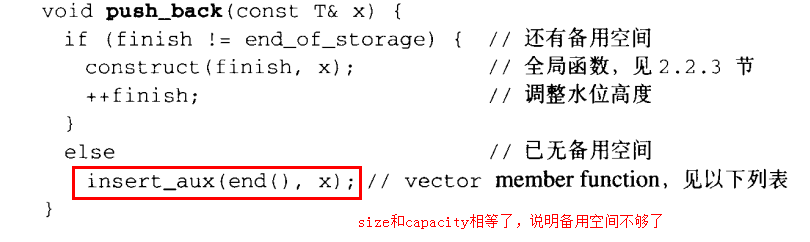在insert_aux里：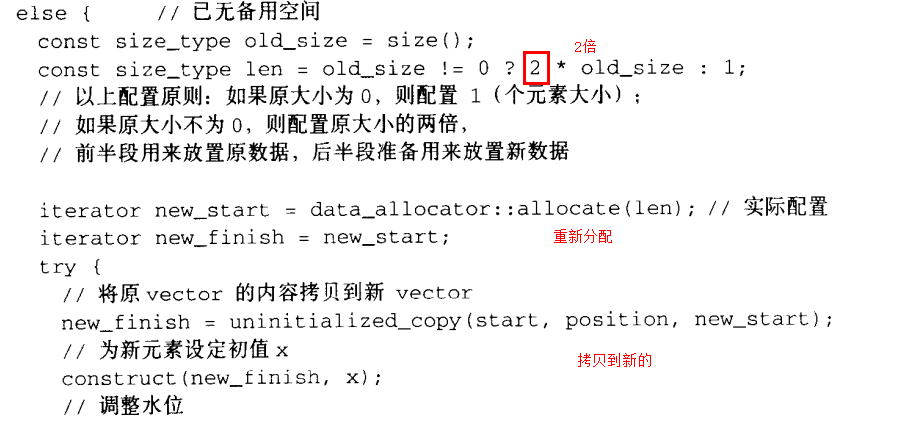这个过程就是容量扩充（备用空间不够了，就重新开辟更大的空间，把旧的拷贝进去，再把旧的释放了，有了更大的新空间，就可以连续的插入和追加元素了）
所以这个过程：重新配置->拷贝数据->释放旧的空间
如果每添加一个元素都这样操作，那效率太低了，索性一次多分分配些空间。
但是注意：
1.vector可以用下标访问元素，但是，只能访问size以内的（比如，size=10，capacity=15,就不能arr这样会报错）
2.一旦扩容，原来空间就已经不存在了，数组首地址也不存在了，因为旧的被复制到新的空间，旧的就被释放了。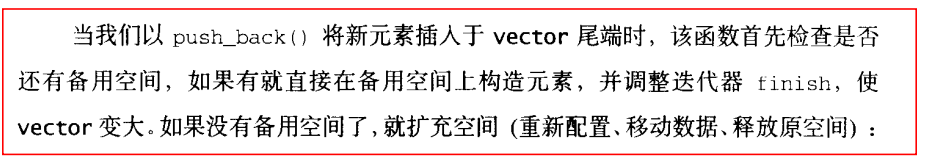下面回归正题：size和capacity有什么区别？
size是真实元素的所占的空间打开，capacity是整个可容纳的空间大小；
如第一个图：
size = finish - start
capacity = end_of_storage - start
下面看一下不同编译器capacity大小分配情况：
这是vs2017的运行结果：
        vector<int> arr(10,1);//10个空间，初值都是1
cout << "size=" << arr.size() << endl;
cout << "capacity=" << arr.capacity() << endl;

arr.push_back(2);//向后面添加一个元素2
cout << "size=" << arr.size() << endl;
cout << "capacity=" << arr.capacity() << endl;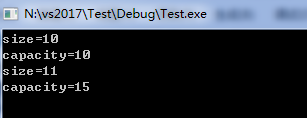这是ubantu16，g++ 5.4的运行结果：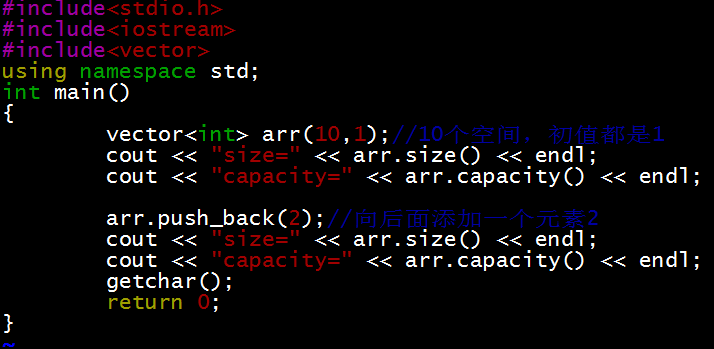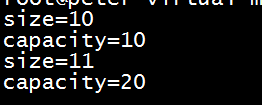为什么capacity不一样呢？
这个编译器有关系，在《STL源码剖析》上可以找到答案：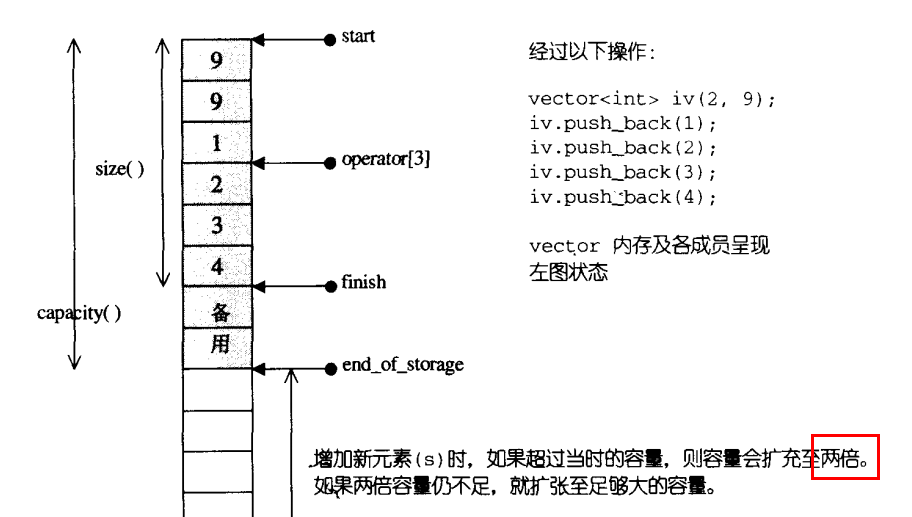但是再vs2017上，capacity在满的时候，扩充的大小为原来的一半，而不是2倍。
===============================分隔线 2019.7.4=====================================================
【问题】怎么使capacity值为0？
先看代码，再讨论
#include "stdafx.h"
#include<vector>
#include<iostream>
void vector_test()
{
std::vector<int> arr(10,1);
std::cout << "arr(10,1):size=" << arr.size() << "; capacity=" << arr.capacity() << std::endl;
arr.push_back(5);
std::cout << "arr.push_back(11):size=" << arr.size() << "; capacity=" << arr.capacity() << std::endl;
arr.clear();
std::cout << "arr.clear():size=" << arr.size() << "; capacity=" << arr.capacity() << std::endl;
//arr.resize(0);
//std::cout << "arr.resize(0):size=" << arr.size() << "; capacity=" << arr.capacity() << std::endl;
//arr.reserve(0);
std::cout << "arr.reserve(0):size=" << arr.size() << "; capacity=" << arr.capacity() << std::endl;
std::vector<int>(arr).swap(arr);

std::cout << "vector<int>(arr).swap(arr):size=" << arr.size() << "; capacity=" << arr.capacity() << std::endl;
}
void Test()
{
vector_test();
}
int main()
{
Test();
getchar();
return 0;
}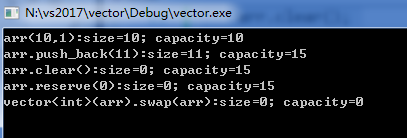1.为什么要把capacity值变为0？
答：当vector容器不断的插入，capacity也不断的扩张，其实capacity是占着内存的，有时候用不了那么多，岂不是很浪费？所以怎么修改capacity的值，也就是我们手动的释放不使用的空间。capacity变为0只是经常会被问到，变为0都会了，变为其他值就是照猫画虎。
2.怎么变？
利用vector的拷贝构造函数。（1）vector的拷贝构造函数，只分配拷贝的元素需要的内存；（2）因为产生临时对象，然后和原来对象进行交换。（3）临时对象消失。
收先验证拷贝构造函数，只分配拷贝的元素需要的内存：
std::vector<int> arr1 = std::vector<int>(arr);
std::cout << "arr1:size=" << arr1.size() << "; capacity=" << arr1.capacity()<<std::endl;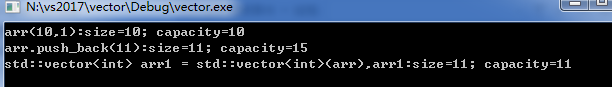然后交换：
交换前需要使用clear，将容器清零。
arr.clear();
std::vector<int> arr1 = std::vector<int>(arr);
arr1.swap(arr);
也可一步到位：
std::vector<int>(arr).swap(arr);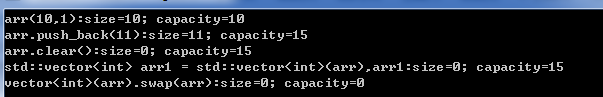另外记录一下resize和reserve这两个函数：
resize是调整size的大小，如果size调整到比原来小，capacity的值不会变化；如果size调整的比原来大，则遵循push_back的扩容原则。
reserve是调整预分配空间的大小，如果调整比原来擦capacity小，依然还是原来capacity的大小。
https://www.cnblogs.com/chailu/p/6179263.html
展开全文• ## C++_vector操作

万次阅读 多人点赞 2019-05-28 11:24:15
vector说明： vector是向量类型，可以容纳许多类型的数据，因此也被称为容器 (可以理解为动态数组，是封装好了的类） vector头文件-vector vector初始化： 方式1. vector<int>a(10);//定义具有10个...


文章目录
1. vector：1.1 vector 说明1.2 vector初始化：1.3 vector对象的常用内置函数使用（举例说明）

2. 顺序访问vector的几种方式，举例说明2.1. 对向量a添加元素的几种方式2.2 从向量中读取元素

3.几个常用的算法4. 写在最后

1. vector：
1.1 vector 说明
vector是向量类型，可以容纳许多类型的数据，因此也被称为容器(可以理解为动态数组，是封装好了的类）进行vector操作前应添加头文件#include <vector>
1.2 vector初始化：
方式1.
//定义具有10个整型元素的向量（尖括号为元素类型名，它可以是任何合法的数据类型），不具有初值，其值不确定
vector<int>a(10);

方式2.
//定义具有10个整型元素的向量，且给出的每个元素初值为1
vector<int>a(10,1);

方式3.
//用向量b给向量a赋值，a的值完全等价于b的值
vector<int>a(b);

方式4.
//将向量b中从0-2（共三个）的元素赋值给a，a的类型为int型
vector<int>a(b.begin(),b.begin+3);

方式5.
 //从数组中获得初值
int b={1,2,3,4,5,6,7};
vector<int> a(b,b+7）;

1.3 vector对象的常用内置函数使用（举例说明）
#include<vector>
vector<int> a,b;
//b为向量，将b的0-2个元素赋值给向量a
a.assign(b.begin(),b.begin()+3);
//a含有4个值为2的元素
a.assign(4,2);
//返回a的最后一个元素
a.back();
//返回a的第一个元素
a.front();
//返回a的第i元素,当且仅当a存在
a[i];
//清空a中的元素
a.clear();
//判断a是否为空，空则返回true，非空则返回false
a.empty();
//删除a向量的最后一个元素
a.pop_back();
//删除a中第一个（从第0个算起）到第二个元素，也就是说删除的元素从a.begin()+1算起（包括它）一直到a.begin()+3（不包括它）结束
a.erase(a.begin()+1,a.begin()+3);
//在a的最后一个向量后插入一个元素，其值为5
a.push_back(5);
//在a的第一个元素（从第0个算起）位置插入数值5,
a.insert(a.begin()+1,5);
//在a的第一个元素（从第0个算起）位置插入3个数，其值都为5
a.insert(a.begin()+1,3,5);
//b为数组，在a的第一个元素（从第0个元素算起）的位置插入b的第三个元素到第5个元素（不包括b+6）
a.insert(a.begin()+1,b+3,b+6);
//返回a中元素的个数
a.size();
//返回a在内存中总共可以容纳的元素个数
a.capacity();
//将a的现有元素个数调整至10个，多则删，少则补，其值随机
a.resize(10);
//将a的现有元素个数调整至10个，多则删，少则补，其值为2
a.resize(10,2);
//将a的容量扩充至100，
a.reserve(100);
//b为向量，将a中的元素和b中的元素整体交换
a.swap(b);
//b为向量，向量的比较操作还有 != >= > <= <
a==b;

2. 顺序访问vector的几种方式，举例说明
2.1. 对向量a添加元素的几种方式
1.向向量a中添加元素
vector<int>a;
for(int i=0;i<10;++i){a.push_back(i);}

2.从数组中选择元素向向量中添加
int a={1,2,3,4,5,6};
vector<int> b;
for(int i=0;i<=4;++i){b.push_back(a[i]);}

3.从现有向量中选择元素向向量中添加
int a={1,2,3,4,5,6};
vector<int>b;
vector<int>c(a,a+4);
for(vector<int>::iterator it=c.begin();it<c.end();++it)
{
b.push_back(*it);
}

4.从文件中读取元素向向量中添加
ifstream in("data.txt");
vector<int>a;
for(int i;in>>i){a.push_back(i);}

5.常见错误赋值方式
vector<int>a;
for(int i=0;i<10;++i){a[i]=i;}//下标只能用来获取已经存在的元素

2.2 从向量中读取元素
1.通过下标方式获取
int a={1,2,3,4,5,6};
vector<int>b(a,a+4);
for(int i=0;i<=b.size()-1;++i){cout<<b[i]<<endl;}

2.通过迭代器方式读取
 int a={1,2,3,4,5,6};
vector<int>b(a,a+4);
for(vector<int>::iterator it=b.begin();it!=b.end();it++){cout<<*it<<"  ";}

3.几个常用的算法
 #include<algorithm>
//对a中的从a.begin()（包括它）到a.end()（不包括它）的元素进行从小到大排列
sort(a.begin(),a.end());
//对a中的从a.begin()（包括它）到a.end()（不包括它）的元素倒置，但不排列，如a中元素为1,3,2,4,倒置后为4,2,3,1
reverse(a.begin(),a.end());
//把a中的从a.begin()（包括它）到a.end()（不包括它）的元素复制到b中，从b.begin()+1的位置（包括它）开始复制，覆盖掉原有元素
copy(a.begin(),a.end(),b.begin()+1);
//在a中的从a.begin()（包括它）到a.end()（不包括它）的元素中查找10，若存在返回其在向量中的位置
find(a.begin(),a.end(),10);

4. 写在最后
本人已经毕业半年有余，深刻感受到生活不易，之前更新博客的时候从没想到自己的博客会有这么多人喜欢，在这里感谢各位朋友的信任，同时也祝福各位同学前程似锦，学业进步!
同时，在这里出售全套的文字学习资料：
具体包括《C primer plus》原文、练习及源代码；C语言具体各部分详解书籍；
《C++ primer plus》原文、练习及源代码；各种C++相关书籍；
《数据结构 C++ 语言描述》《数据结构 C 语言描述》《数据结构》严蔚敏版；数据结构相关书籍；
《powershell 入门教程》；《剑指offer 第二版》原文及源代码；《深入了解计算机系统》中文版，布莱恩特著，等等书籍；
全套资料售价￥68.8，单套￥19.9
展开全文• ## Vector

千次阅读 多人点赞 2018-03-17 14:08:58
Vector Vector非常类似ArrayList，都继承自AbstractList并且都实现了List接口，但是Vector是同步的。由Vector创建的Iterator，虽然和ArrayList创建的Iterator是同一接口，但是，因为Vector是同步的，当一个Iterator...
Vector
Vector非常类似ArrayList，都继承自AbstractList并且都实现了List接口，但是Vector是同步的。由Vector创建的Iterator，虽然和ArrayList创建的Iterator是同一接口，但是，因为Vector是同步的，当一个Iterator被创建而且正在被使用，另一个线程改变了Vector的状态（例如，添加或删除了一些元素），这时调用Iterator的方法时将抛出ConcurrentModificationException，因此必须捕获该异常。
Vector实现List接口，所以里面的元素是有序的。
vector.elements()方法返回一个枚举类型，我们可以用来遍历整个vector。
vector.iterator()方法返回一个迭代器，同样可以用来遍历整个vector
vector.contains(E element)方法，如果vector中存在指定元素则返回true，否则false。
vector.get(int index)方法，返回指定位置的元素，如果超过vector的capacity则报错。
初始化vector时，默认不带参数的话，vector的初始capacity大小为10。当当前空间不足以加入新元素时，capacity会加倍，即从10变成20，40，80......
当然我们也可以使用下面两种带参数的构造
1.Vector vector=new Vector(int  capacity);指定初始化大小，不允许负数大小的初始化。如果初始化大小为0，则加入数据时大小变为1，然后每次扩充遵循加倍原则。
2.Vector vector=new Vector(int capacity,int increasesize)指定初始化大小，并且以后每次容量不足时，大小每次增加increasesize。

import java.util.Enumeration;
import java.util.Iterator;
import java.util.Vector;

public class Test {
public static void main(String[] args){
Vector vector=new Vector(0);
System.out.println("vector的初始大小： "+vector.capacity());
vector.add("大家好"); //Appends the specified element to the end of this Vector.
Enumeration e=vector.elements();//Returns an enumeration of the components of this vector.
System.out.println(vector.capacity());
while(e.hasMoreElements()){
System.out.println(e.nextElement());
}
System.out.println("---------分界线----------------------");
Iterator iterator=vector.iterator(); //使用迭代器
while(iterator.hasNext()){
System.out.println(iterator.next());
}
for(int i=0;i<100;i++) {
System.out.print(vector.capacity()+" ");
if(i%10==0) System.out.println();
}
System.out.println(vector.capacity());
System.out.println(vector.contains("你好"));
System.out.println(vector.get(2));
}
}

运行结果：
vector的初始大小： 10
10
大家好
111
222
你好
---------分界线----------------------
大家好
111
我在第三位
222
你好
10
10 10 10 10 20 20 20 20 20 20
20 20 20 20 40 40 40 40 40 40
40 40 40 40 40 40 40 40 40 40
40 40 40 40 80 80 80 80 80 80
80 80 80 80 80 80 80 80 80 80
80 80 80 80 80 80 80 80 80 80
80 80 80 80 80 80 80 80 80 80
80 80 80 80 160 160 160 160 160 160
160 160 160 160 160 160 160 160 160 160
160 160 160 160 160 160 160 160 160 160
true
我在第三位
初始化大小为0
vector的初始大小： 0
capacity：1
capacity：2
capacity：4
capacity：4
大家好
111
222
你好
8 8 8 8 16 16 16 16 16 16
16 16 32 32 32 32 32 32 32 32
32 32 32 32 32 32 32 32 64 64
64 64 64 64 64 64 64 64 64 64
64 64 64 64 64 64 64 64 64 64
64 64 64 64 64 64 64 64 64 64
128 128 128 128 128 128 128 128 128 128
128 128 128 128 128 128 128 128 128 128
128 128 128 128 128 128 128 128 128 128
128 128 128 128 128 128 128 128 128 128
128
true
222


展开全文• C++ vector类为内置数组提供了一种替代表示，与string类一样 vector 类是随标准 C++引入的标准库的一部分，使用时需包含头文件： #include <vector> 二、使用方式 C++ vector类有两种使用方式： 第一种：...
一、介绍
C++ vector类为内置数组提供了一种替代表示，与string类一样 vector 类是随标准 C++引入的标准库的一部分，使用时需包含头文件：
#include <vector>
二、使用方式
C++ vector类有两种使用方式：
第一种：STL方式
vector< string > text;
1.  我们向 vector 中插入元素，而不再是索引元素，以及向元素赋值，例如 push_back()操作，就是在 vector 的后面插入一个元素下面的 while 循环从标准输入读入一个字符串序列并每次将一个字符串插入到 vector 中
string word;
while ( cin >> word ) {
text.push_back( word );
// ...
}
虽然我们仍可以用下标操作符来迭代访问元素
cout << "words read are: \n";

for ( int ix = 0; ix < text.size(); ++ix )
cout << text[ ix ] << ' ';

cout << endl; 
但是 更典型的做法是使用vector 操作集中的begin()和 end()所返回的迭代器 iterator   对 ：
cout << "words read are: \n";

for ( vector<string>::iterator it = text.begin();
it != text.end(); ++it )
cout << *it<< ' ';

cout << endl 
iterator 是标准库中的类，它具有指针的功能
*it;  对迭代器解引用，并访问其指向的实际对象   ++it;
向前移动迭代器 it 使其指向下一个元素
2.  注意 不要混用这两种习惯用法， 例如，下面的定义   vector< int > ivec;  定义了一个空vector 再写这样的语句   ivec[ 0 ] = 1024;  就是错误的 ，因为 ivec 还没有第一个元素，我们只能索引 vector 中已经存在的元素 size()操作返回 vector 包含的元素的个数。
3. 类似地 当我们用一个给定的大小定义一个vector 时，例如  ： vector<int> ia( 10 );  任何一个插入操作都将增加vector 的大小，而不是覆盖掉某个现有的元素，这看起来好像是很显然的，但是下面的错误在初学者中并不少见：
const int size = 7;
int ia[ size ] = { 0, 1, 1, 2, 3, 5, 8 };
vector< int > ivec( size );
for ( int ix = 0; ix < size; ++ix )
ivec.push_back( ia[ ix ]); 
程序结束时ivec 包含 14 个元素, ia 的元素从第八个元素开始插入。
第二种：类数组使用
1. 定义一个已知长度的 vector ：
vector< int > ivec( 10 );  //类似数组定义int ia[10 ];
可以通过ivec[索引号] 来访问元素
使用 if ( ivec.empty() ) 判断是否是空，ivec.size()判断元素个数。

2. vector的元素被初始化为与其类型相关的缺省值：算术和指针类型的缺省值是 0，对于class 类型，缺省值可通过调用这类的缺省构造函数获得，我们还可以为每个元素提供一个显式的初始值来完成初始化，例如   vector< int > ivec( 10, -1 );  定义了 ivec 它包含十个int型的元素每个元素都被初始化为-1
对于内置数组我们可以显式地把数组的元素初始化为一组常量值，例如：  int ia[ 6 ] = { -2, -1, 0, 1, 2, 1024 };
我们不能用同样的方法显式地初始化 vector ，但是可以将 vector 初始化为一个已有数组的全部或一部分，只需指定希望被用来初始化 vector 的数组的开始地址以及数组最末元的下一位置来实现，例如：   // 把 ia 的 6 个元素拷贝到 ivec 中  vector< int > ivec( ia, ia+6 );
被传递给ivec 的两个指针标记了用来初始化对象的值的范围，第二个指针总是指向要拷贝的末元素的下一位置，标记出来的元素范围也可以是数组的一个子集，例如 :
// 拷贝 3 个元素 ia,ia, ia  vector< int > ivec( &ia[ 2 ], &ia[ 5 ] );
3. 与内置数组不同 vector 可以被另一个 vector 初始化或被赋给另一个 vector 例如
vector< string > svec;
void init_and_assign()
{
// 用另一个 vector 初始化一个 vector
vector< string > user_names( svec );
// ...

// 把一个 vector 拷贝给另一个 vector
svec = user_names;
}
三、vector使用总结：
1. vector的初始化：
可以有五种方式,举例说明如下：
（1） vector<int> a(10); //定义了10个整型元素的向量（尖括号中为元素类型名，它可以是任何合法的数据类型），但没有给出初值，其值是不确定的。 （2）vector<int>a(10,1); //定义了10个整型元素的向量,且给出每个元素的初值为1 （3）vector<int>a(b); //用b向量来创建a向量，整体复制性赋值 （4）vector<int>a(b.begin(),b.begin+3); //定义了a值为b中第0个到第2个（共3个）元素 （5）intb={1,2,3,4,5,9,8};vector<int> a(b,b+7); //从数组中获得初值
2. vector对象的几个重要操作
举例说明如下：
（1）a.assign(b.begin(), b.begin()+3);//b为向量，将b的0~2个元素构成的向量赋给a （2）a.assign(4,2);//是a只含4个元素，且每个元素为2 （3）a.back();//返回a的最后一个元素 （4）a.front();//返回a的第一个元素 （5）a[i]; //返回a的第i个元素，当且仅当a[i]存在2013-12-07 （6）a.clear();//清空a中的元素 （7）a.empty();//判断a是否为空，空则返回ture,不空则返回false （8）a.pop_back();//删除a向量的最后一个元素 （9）a.erase(a.begin()+1,a.begin()+3);//删除a中第1个（从第0个算起）到第2个元素，也就是说删除的元素从a.begin()+1算起（包括它）一直到a.begin()+3（不包括它） （10）a.push_back(5);//在a的最后一个向量后插入一个元素，其值为5 （11）a.insert(a.begin()+1,5);//在a的第1个元素（从第0个算起）的位置插入数值5，如a为1,2,3,4，插入元素后为1,5,2,3,4 （12）a.insert(a.begin()+1,3,5);//在a的第1个元素（从第0个算起）的位置插入3个数，其值都为5 （13）a.insert(a.begin()+1,b+3,b+6);//b为数组，在a的第1个元素（从第0个算起）的位置插入b的第3个元素到第5个元素（不包括b+6），如b为1,2,3,4,5,9,8，插入元素后为1,4,5,9,2,3,4,5,9,8 （14）a.size();//返回a中元素的个数； （15）a.capacity();//返回a在内存中总共可以容纳的元素个数 （16）a.rezize(10);//将a的现有元素个数调至10个，多则删，少则补，其值随机 （17）a.rezize(10,2);//将a的现有元素个数调至10个，多则删，少则补，其值为2 （18）a.reserve(100);//将a的容量（capacity）扩充至100，也就是说现在测试a.capacity();的时候返回值是100.这种操作只有在需要给a添加大量数据的时候才 显得有意义，因为这将避免内存多次容量扩充操作（当a的容量不足时电脑会自动扩容，当然这必然降低性能）  （19）a.swap(b);//b为向量，将a中的元素和b中的元素进行整体性交换 （20）a==b; //b为向量，向量的比较操作还有!=,>=,<=,>,<
3. 顺序访问vector的几种方式
举例说明如下： 1、向向量a中添加元素
vector<int> a;
for(int i=0;i<10;i++)
a.push_back(i);
2、也可以从数组中选择元素向向量中添加
int a={1,2,3,4,5,6};
vector<int> b；
for(int i=1;i<=4;i++)
b.push_back(a[i]);
3、也可以从现有向量中选择元素向向量中添加
int a={1,2,3,4,5,6};
vector<int> b;
vector<int> c(a,a+4);
for(vector<int>::iterator it=c.begin();it<c.end();it++)
b.push_back(*it);
4、也可以从文件中读取元素向向量中添加
ifstream in("data.txt");
vector<int> a;
for(int i; in>>i)
a.push_back(i);
5、易犯错误
vector<int> a;
for(int i=0;i<10;i++)
a[i]=i;
//这种做法以及类似的做法都是错误的。下标只能用于获取已存在的元素，而现在的a[i]还是空的对象
（2）从向量中读取元素 1、通过下标方式读取
int a={1,2,3,4,5,6};
vector<int> b(a,a+4);
for(vector<int>::iterator it=b.begin();it!=b.end();it++)
cout<<*it<<" ";
四、几种重要的算法
使用时需要包含头文件： #include<algorithm> （1）sort(a.begin(),a.end()); //对a中的从a.begin()（包括它）到a.end()（不包括它）的元素进行从小到大排列 （2）reverse(a.begin(),a.end()); //对a中的从a.begin()（包括它）到a.end()（不包括它）的元素倒置，但不排列，如a中元素为1,3,2,4,倒置后为4,2,3,1 （3）copy(a.begin(),a.end(),b.begin()+1); //把a中的从a.begin()（包括它）到a.end()（不包括它）的元素复制到b中，从b.begin()+1的位置（包括它）开始复制，覆盖掉原有元素 （4）find(a.begin(),a.end(),10); //在a中的从a.begin()（包括它）到a.end()（不包括它）的元素中查找10，若存在返回其在向量中的位置
五、其它使用举例：
（1）把一个vector中元素追加到另外一个容器vector，示例程序如下：
#include <vector>

#include <iostream>

template <typename type> void printvector(std::vector<type> const &v, std::ostream &os = std::cout)

{

for (typename std::vector<type>::size_type sz = 0; sz != v.size(); ++sz)

os << v[sz] << " ";

os << std::endl;

}

int main()

{

int a = {1, 2, 3, 4, 5};

std::vector<int> v1(a, a + 3), v2(a + 3, a + 5);

printvector(v1); // 结果是1 2 3

printvector(v2); // 结果是4 5

v1.insert(v1.end(), v2.begin(), v2.end()); // 把v2加到v1末尾

printvector(v1); // 结果是1 2 3 4 5

return 0;

}
（2）类和结构体存vector入实例:由于vector只允许一个占位，所以才将struct塞进vector，以弥补vector的不足。
#include "stdafx.h"
#include <vector>
#include <string>
using namespace std;
class AClass
{
public:
int num;
string name;
};
struct AStruct
{
int num;
string name;
};
void TestStruct()
{
//类的使用
AClass Ac;
vector<AClass> vc;
Ac.num=10;
Ac.name="name";
vc.push_back(Ac);
AClass d;
for (vector<AClass>::iterator it=vc.begin();it<vc.end();++it)
{
d=*it;
cout<<d.num<<endl;
}
//结构体的使用
AStruct As;
vector<AStruct> vs;
As.num=10;
As.name="name";
vs.push_back(As);
AStruct ds;
for (vector<AStruct>::iterator it=vs.begin();it<vs.end();++it)
{
ds=*it;
cout<<ds.num<<endl;
}
}
void TestPoint()
{
//类的使用
AClass *Ac=new AClass;
vector<AClass *> vc;
Ac->num=10;
Ac->name="name";
vc.push_back(Ac);
AClass *d;
for (vector<AClass*>::iterator it=vc.begin();it<vc.end();++it)
{
d=*it;
cout<<d->num<<endl;
}
}
int _tmain(int argc, _TCHAR* argv[])
{
TestStruct();
TestPoint();
int n;
cin>>n;
return 0;
}
（3）C++中将两个vector中的值整合到另一个vector中，vecB和vecA中有相同的struct个数，现在想将每个vecA中的每个a的值传给vecC中c1，每个vecB中的每个b的值传给vecC中c2，也就是将两个容器中的内容整合到新的容器C中
struct A
{
int a;
};
vector<A> vecA;
struct B
{
int b;
};
vector<B> vecB;
struct C
{
int c1;
int c2;
};
vector<C> vecC;
容器C和A、B中元素类型不同，迭代器类型就不同，所以不能用容器算法，使用迭代器遍历赋值，合并代码如下：
第一种方法：
for(vecA::const_iterator itA = vecA.begin(), VecB::const_iterator itB = vecB.begin();
itA != vecA.end() && itB != vecB.end(); itA++, itB++){
C c;
c.c1 = (*itA).a;
c.c2 = (*itB).b;
vecC.push_back(c);
}
第二种方法：
void MergeVector(vector<A> &vectorA,vector<B> &vectorB,vector<C> &vectorC)
{
vector<A>::iterator pva;
vector<B>::iterator pvb;
vector<C>::iterator pvc;
pva = vectorA.begin();
pvb = vectorB.begin();
pvc = vectorC.begin();
while(pva!=vectorA.end())
{
*pvc->c1 = *pva->a;
*pvc->c2 = *pvb->b;
pva++;
pvb++;
pvc++;
}
}
（4）建立两个int类型的向量vector,利用merge算法合并，再用sort算法对合并后算法排序
#include <iostream>
#include <vector>
#include <algorithm>
using namespace std;
vector<int> merge(vector<int> ,vector<int> );
int main()
{
vector<int> v1;
v1.push_back(4);
v1.push_back(6);
v1.push_back(2);
vector<int> v2;
v2.push_back(3);
v2.push_back(1);
v2.push_back(5);
vector<int> v3=merge(v1,v2);
sort(v3.begin(),v3.end());
for(vector<int>::iterator it=v3.begin();it!=v3.end();++it){
cout<<*it<<endl;
}
}
vector<int> merge(vector<int> v1,vector<int> v2)
{
v1.insert(v1.end(),v2.begin(),v2.end());
return v1;
}
转自：https://blog.csdn.net/fanyun_01/article/details/56842637
展开全文• ## C++vector的使用总结及常用vector操作

万次阅读 多人点赞 2018-07-12 22:10:22
一、C++ vector类为内置数组提供了一种替代表示，与string类一样 vector 类是随标准 C++引入的标准库的一部分，使用时需包含头文件： #include &lt;vector&gt; 二、C++ vector类有两种使用方式： 第一种...C++ 容器
• 参考链接： C++ Vectorcapacity与size vector中这两个属性很容易弄混淆。 size是当前vector容器真实占用的大小，也就是容器当前拥有多少个容器。 capacity是指在发生realloc前能允许的最大元素数，即预分配的...
• 在介绍resize()，reserve()，size()和capacity()函数之前，先简单介绍一下c++中vector的概念。 vector：顺序容器（可变大小数组）。支持快速随机访问。在尾部之外的位置插入或删除元素可能很慢。 既然vector是个...C++
• 1.概念 容器的capacity：当前分配的存储容量，其实也就是当前情况下能够存储的元素个数 ...=vector.capacity(),一旦元素个数超过了当点分配空间容许的元素个数，容器就会扩容。 3.验证 #include<iostr数据结构
• 简单而言，size（）指的是当前Vector中存放元素的大小，即他当前存放了多少个元素，capacity()指的是他能够存放多少个元素。 两个方法可以控制存放元素的大小和最大容纳数量 reserve可以保证容纳的大小 resize()可以...
• vector对象是如何增长的(第五版P356)       ~~~~~~      为了支持快速随机访问，vector将元素连续存储–每个元素紧挨着前一个元素存储。   ...
• vector是系统内部管理其长度的。 其默认规则是，先申请一片内存，当需要增加元素时，如果元素个数超过已有内存可存放的个数时，再申请一批内存。 这里很明显就有了两个长度，一个是元素个数，一个是可以存放元素的...
• 今天测试了一下vector大小和容量的问题。 size 是实际上存储了多少个元素。 capacity是留了多少的空间。 如果size增加到和capacity相等的时候，下一次再添加元素的时候，capaticy会变大许多。 同时，初始化5是...c++
• vector中这两个属性很容易弄混淆。 size是当前vector内变量真实占用的大小。 capacity是预留的空间大小。C++ STL
• capacity:向量的容量，可能大于或等于向量实际的长度 size:向量实际的容量，是多少就是多少 max_size:向量实际可以存放下的最大空间 ...vector> //返回分配的存储容量大小 //返回当前分配给向量的存...
• 前言STL容器的内存分配是一个重点内容，不同版本的STL的内存分配策略不尽相同，下面我从2个测试例子来说明常用的2个版本的STL中vector内存分配的规律。2. windows环境 说明：编译器是visual s内存分配 STL C++
• vector中的size（）函数返回当前数组的元素...capacity是返回数组的大小，跟插入的数量有关，vector的容量size是比其数组size第一个大的二进制数。 即可能的capacity值为 1 2 4 8 . . . 1024 2048 4096 ...
• vector 的 size 达到 capacity 时，当前 vector 预留的连续内存已经完全写满，此时如果通过 push_back 进行插入操作时，会导致该 vector 进行扩展。 扩展前后的变化 扩展后，capacity 会增大 扩展时会...
• vectorcapacity是指最少要多少元素才会使容器重新分配，...乍一看，如果没有没有使用reserve()的话，vector的size和capacity应该是相同的，但是不见得。 代码1 int m,n; n=obstacleGrid.capacity(); m=obstacl...
• vector::capacity（容量） Return size of allocated storage capacity Returns the size of the storage space currently allocated for the vector, expressed in terms of elements. This capacity...
• 要注意的是capacity并不是vector所支持的最大容量，当容量被耗尽或者是需要比容量更多的空间时，vector会自动通过重新分配存储空间来完成增长。理论上最大的容量可以从成员方法max_size中获得。 The  ...STL
• vector3Capacity(内存容量)1.size2.max_size3.resize4.capacity5.reserve6.shrink_to_fit Capacity(内存容量) 1.size 用来看内部有多少个字节 struct A{ A(int a = 1, int b = 2) :_a(a) , _b(b) {} int...c++
• 主要介绍了vector 容器大小相关的操作函数（size、max_szie、capacity），同时介绍了容器大小超出容器capacity能力会内存重新分配以及iterator失效简单分析。同时给出了避免内存重新分配的两种方法。size max_size
• vectorcapacity和size属性区别 vector中这两个属性很容易弄混淆。 size是当前vector容器真实占用的大小，也就是容器当前拥有多少个容器。 capacity是指在发生realloc前能允许的最大元素数，即预分配的内存空间...
• 前言 C++STL中的vector有三个与容器大小相关的方法 size max_size capacity 它们看着相似，实则...capacity方法返回的是当前程序已经分配给该vector对象的内存大小（单位为元素个数）。 3. max_size max_size方法c++ 数据结构 stl
• 1. 属性size是容器里真实个数； 2. 属性capacity是realloc预分配的值； 详见：https://blog.csdn.net/u013575812/article/details/51171135...